A ball is thrown upward with a certain speed. It passes through the same point at 3 second and 7 second from the start. The maximum height achieved by the ball is:

1.  500 m

2.  250 m

3.  125 m

4.  450 m

Subtopic:  Uniformly Accelerated Motion |
To view explanation, please take trial in the course below.
NEET 2022 - Target Batch - Aryan Raj Singh
To view explanation, please take trial in the course below.
NEET 2022 - Target Batch - Aryan Raj Singh

Difficulty Level:

For the following acceleration versus time graph the corresponding velocity versus displacement graph is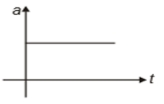1.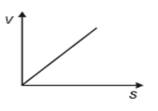2.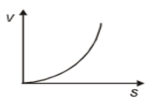3.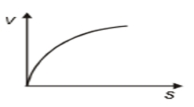4.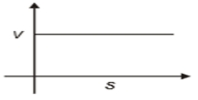Subtopic:  Uniformly Accelerated Motion |
To view explanation, please take trial in the course below.
NEET 2022 - Target Batch - Aryan Raj Singh
To view explanation, please take trial in the course below.
NEET 2022 - Target Batch - Aryan Raj Singh

Difficulty Level:

The displacement x of a particle moving in one dimension under the action of the constant force is related to time t by the equation , where x is in meters and t is in seconds. What is the displacement of the particle from t = 0 s to t = 6 s?

1.  0

2.  12 m

3.  6 m

4.  18 m

Subtopic:  Distance & Displacement |
To view explanation, please take trial in the course below.
NEET 2022 - Target Batch - Aryan Raj Singh
To view explanation, please take trial in the course below.
NEET 2022 - Target Batch - Aryan Raj Singh

Difficulty Level:

The acceleration a (in ${\mathrm{ms}}^{-2}$) of a body, starting from rest varies with time t(in s) following the equation a = 3t+4. The velocity of the body at time t = 2s will be :

1. 10 ${\mathrm{ms}}^{-1}$

2. 18 ${\mathrm{ms}}^{-1}$

3. 14 ${\mathrm{ms}}^{-1}$

4. 26 ${\mathrm{ms}}^{-1}$

Subtopic:  Non Uniform Acceleration |
To view explanation, please take trial in the course below.
NEET 2022 - Target Batch - Aryan Raj Singh
To view explanation, please take trial in the course below.
NEET 2022 - Target Batch - Aryan Raj Singh

Difficulty Level:

A body thrown vertically so as to reach its maximum height in t second. The total time from the time of projection to reach a point at half of its maximum height while returning (in second) is:

1. $\sqrt{2}t$

2. $\left(1+\frac{1}{\sqrt{2}}\right)t$

3. $\frac{3t}{2}$

4. $\frac{t}{\sqrt{2}}$

Subtopic:  Uniformly Accelerated Motion |
To view explanation, please take trial in the course below.
NEET 2022 - Target Batch - Aryan Raj Singh
To view explanation, please take trial in the course below.
NEET 2022 - Target Batch - Aryan Raj Singh

Difficulty Level:

A stone falls freely from rest from a height h and it travels a distance $\frac{9h}{25}$ in the last second. The value of h is:

1. 145 m

2. 100 m

3. 125 m

4. 200 ms

Subtopic:  Uniformly Accelerated Motion |
To view explanation, please take trial in the course below.
NEET 2022 - Target Batch - Aryan Raj Singh
To view explanation, please take trial in the course below.
NEET 2022 - Target Batch - Aryan Raj Singh

Difficulty Level:

A point moves in a straight line under the retardation a${\mathrm{v}}^{2}$. If the initial velocity is u, the distance covered in 't' seconds is-

1.  $\mathrm{aut}$

2.

3.

4.

Subtopic:  Non Uniform Acceleration |
To view explanation, please take trial in the course below.
NEET 2022 - Target Batch - Aryan Raj Singh
To view explanation, please take trial in the course below.
NEET 2022 - Target Batch - Aryan Raj Singh

Difficulty Level:

A particle is thrown upwards from ground. It experiences a constant resistance force which can produce retardation of 2 $m/{s}^{2}$. The ratio of time of ascent to the time of descent is:

(1) 1:1

(2) $\sqrt{\frac{2}{3}}$

(3) $\frac{2}{3}$

(4) $\sqrt{\frac{3}{2}}$

Subtopic:  Uniformly Accelerated Motion |
To view explanation, please take trial in the course below.
NEET 2022 - Target Batch - Aryan Raj Singh
To view explanation, please take trial in the course below.
NEET 2022 - Target Batch - Aryan Raj Singh

Difficulty Level:

A bullet loses $\frac{1}{20}$ of its velocity passing through a plank. The least number of planks required to stop the bullet is (All planks offers same retardation)

(1) 10

(2) 11

(3) 12

(4) 23

Subtopic:  Uniformly Accelerated Motion |
To view explanation, please take trial in the course below.
NEET 2022 - Target Batch - Aryan Raj Singh
To view explanation, please take trial in the course below.
NEET 2022 - Target Batch - Aryan Raj Singh

Difficulty Level:

A body starts from the origin and moves along the X-axis such that the velocity at any instant is given by $\left(4{t}^{3}-2t\right)$, where t is in sec and velocity in m/s. What is the acceleration of the particle, when it is 2 m from the origin ?

1. 28 m/s2

2. 22 m/s2

3. 12 m/s2

4. 10 m/s2

Subtopic:  Non Uniform Acceleration |
To view explanation, please take trial in the course below.
NEET 2022 - Target Batch - Aryan Raj Singh
To view explanation, please take trial in the course below.
NEET 2022 - Target Batch - Aryan Raj Singh

Difficulty Level: